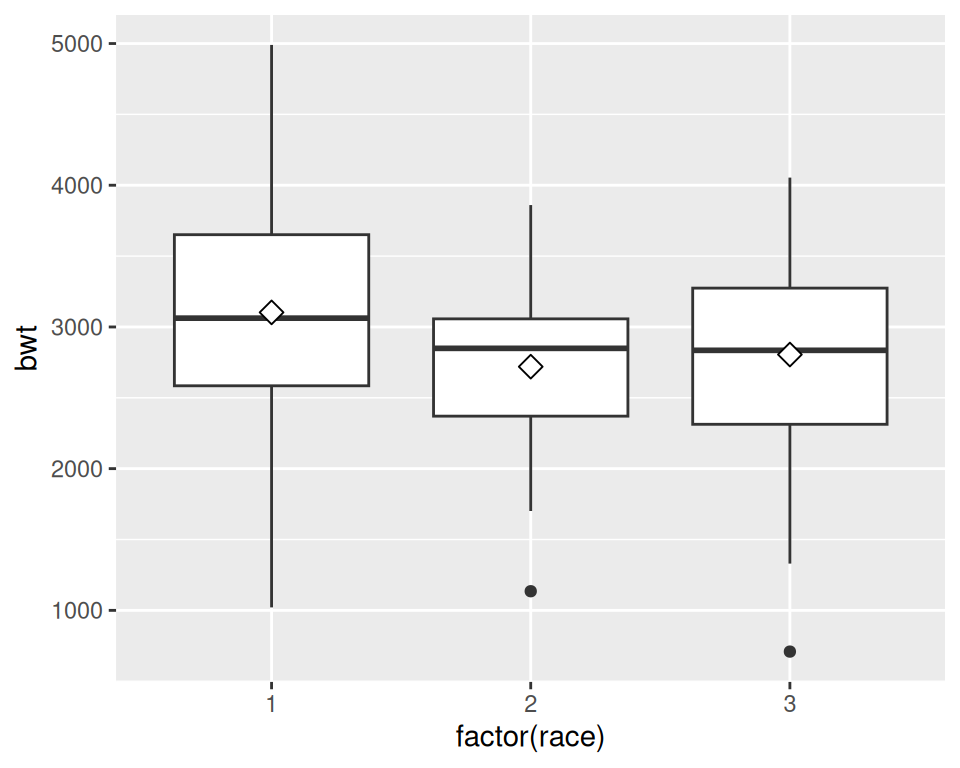## 6.8 Adding Means to a Box Plot

### 6.8.1 Problem

You want to add markers for the mean to a box plot.

### 6.8.2 Solution

Use `stat_summary()`. The mean is often shown with a diamond, so we’ll use shape 23 with a white fill. We’ll also make the diamond slightly larger by setting `size = 3` (Figure 6.21):

``````library(MASS) # Load MASS for the birthwt data set

ggplot(birthwt, aes(x = factor(race), y = bwt)) +
geom_boxplot() +
stat_summary(fun.y = "mean", geom = "point", shape = 23, size = 3, fill = "white")
#> Warning: The `fun.y` argument of `stat_summary()` is deprecated as of ggplot2 3.3.0.